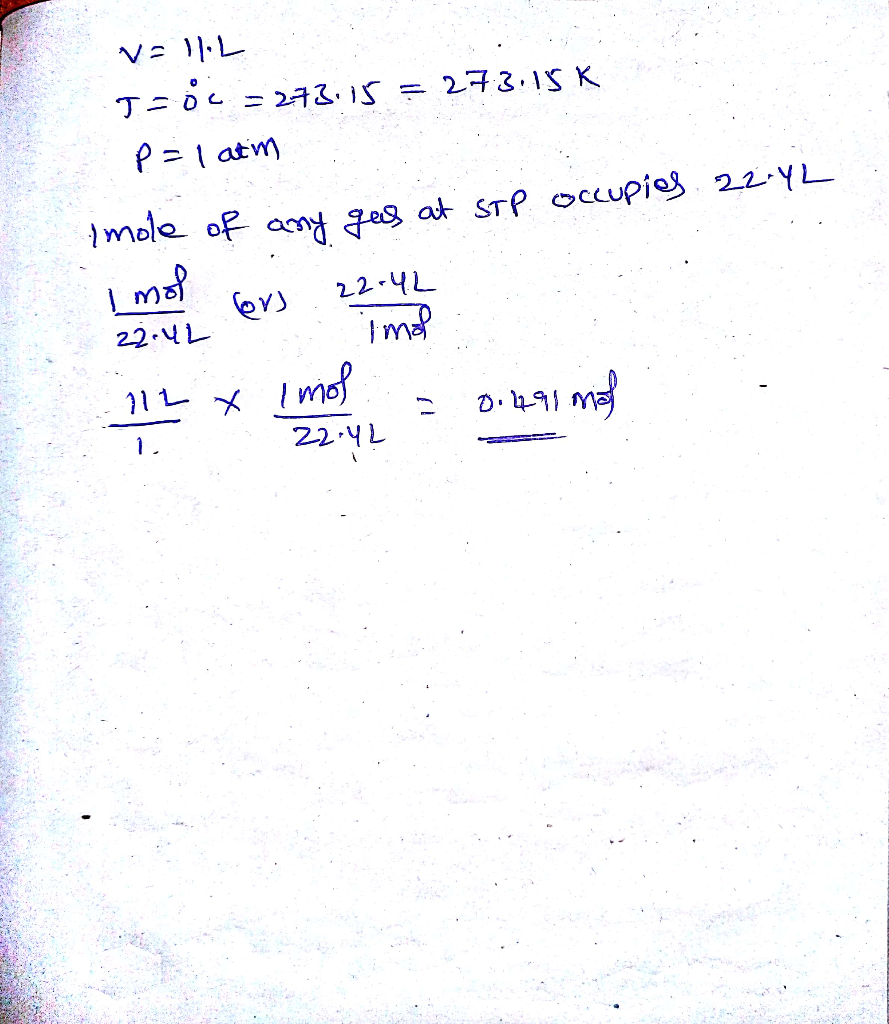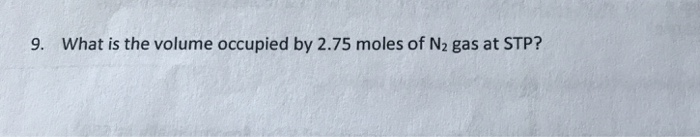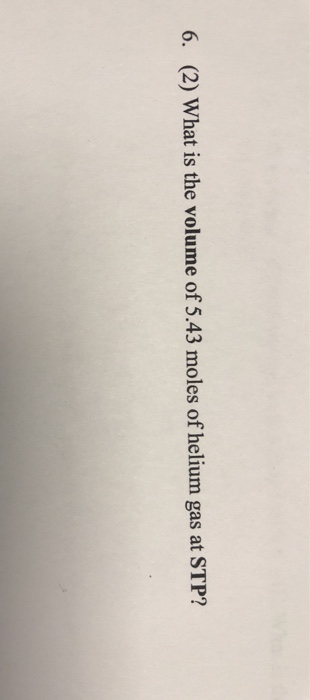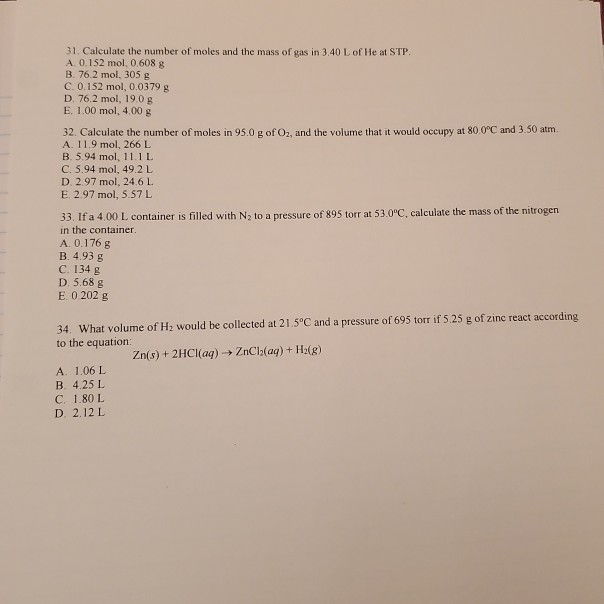Question

Calculate the number of moles of H2 in 11L of gas at STP.#### Earn Coins

Coins can be redeemed for fabulous gifts.

Similar Homework Help Questions
• ### Calculate (a) the number of moles in 1.00m^3 of gas at STP, and (b) the number...

Calculate (a) the number of moles in 1.00m^3 of gas at STP, and (b) the number of liters of gas per mole.

• ### Calculate the number of moles of hydrogen gas in the eudiometer if 24.99mL of H2 exert...

Calculate the number of moles of hydrogen gas in the eudiometer if 24.99mL of H2 exert a pressure of 567 torr at 296.2K.

• ### Consider the following gas reaction at STP: H2(g) +O2(g) -->H2O2(g). Calculate the final volume if 2.0...

Consider the following gas reaction at STP: H2(g) +O2(g) -->H2O2(g). Calculate the final volume if 2.0 moles of hydrogen gas react with 2.0 moles of oxygen gas. (R=0.08206 L*atm/mol*K)

• ### Calculate the number of moles of O2 in 2.27 LL of O2 gas. Calculate the volume,...

Calculate the number of moles of O2 in 2.27 LL of O2 gas. Calculate the volume, in liters, occupied by 1.46 moles of N2 gas. Calculate the volume, in liters, occupied by 65.1 g of Ar gas. Calculate the number of grams of H2 in 1610 mL of H2 gas.

• ### Use molar volune to calculate each of the following at STP: a.) the volume, in liters, occupied. y 2.70 moles of N2 gas...

Use molar volune to calculate each of the following at STP: a.) the volume, in liters, occupied. y 2.70 moles of N2 gas b.) the number of moles of CO2 in 2.90 L of CO2 gas

• ### If you find that (3.00x10^-3) moles of H2 gas have a volume of (1.370x10^-1) L under...

If you find that (3.00x10^-3) moles of H2 gas have a volume of (1.370x10^-1) L under STP conditions, what is the volume of 1 mol under the same conditions?

• ### 9. What is the volume occupied by 2.75 moles of N2 gas at STP?9. What is the volume occupied by 2.75 moles of N2 gas at STP?

• ### 6. (2) What is the volume of 5.43 moles of helium gas at STP?6. (2) What is the volume of 5.43 moles of helium gas at STP?

• ### answer all please 31. Calculate the number of moles and the mass of gas in 3.40...answer all please 31. Calculate the number of moles and the mass of gas in 3.40 L of He at STP. A. 0.152 mol 0.608 g B. 76.2 mol. 305 g C. 0.152 mol, 0.0379 g D. 76.2 mol. 19.0 g E. 1.00 mol, 4.00 g 32. Calculate the number of moles in 95.0 g of O., and the volume that it would occupy at 80.0°C and 3.50 atm A 11.9 mol, 266 L B. 5.94 mol, 11.1 L C....

• ### Calculate the number of moles of HI that are at equilibrium with 1.42 mol of H2...

Calculate the number of moles of HI that are at equilibrium with 1.42 mol of H2 and 1.42 mol of I2 in a 3.50 L flask at 450.°C. H2(g) + I2(g) equilibrium reaction arrow 2 HI(g) Kc = 65.7 at 450.°C